# 词法分析

### Prerequist Knowledge

• Automata Theory
• Finite State Machine
• Formal Language
• Regular Language
• Context Free Grammar
• DFA / NFA
• Lexcial Analysis
• Algorithms
• Recursion
• Tools

## DFA

1. 一个确定的状态集合，用 $Q$ 表示
2. 一组输入的字符，用 $\sum$ 表示
3. 一个状态转移函数（正则表达式），用 $\delta$ 表示
4. 一个初始状态，用 $q_0$ 表示，$q_0$ 属于 $Q$ 的一部分
5. 一组最终状态(Final State)，用 $F$ 表示，$F \subseteq Q$，也可以叫Accepting State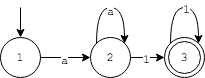#定义状态转义函数
edges = {
(1,'a') : 2, #state#1 takes an input of 'a', transfer the state to #2
(2,'a') : 2,
(2,'1') : 3,
(3,'1') : 3
}
#定义状态机的结束状态，可能有多个结束状态，用array表示
accepting = 

#string: 输入字符
#current: 当前状态/初始状态
#edges: 状态转移方程
#accepting: 最终状态集合
def fsmsim(string, current, edges, accepting):
if string == "":
#递归基，如果当前字符处于Accepting State则终止递归，匹配结束
return current in accepting
else:
letter = string
key = (current,letter)
#进入状态机
if key in edges:
next_state = edges[key]
remaining_string = string[1:]
#递归
return fsmsim(remaining_string,next_state,edges,accepting)
else:
return False;

#test - case:
print(fsmsim("aaa111",1,edge,accepting)) #=>True
print(fsmsim("a1a1a1",1,edge,accepting)) #=>Flase
print(fsmsim("",1,edge,accepting)) #=>False


1. 由正则式构造出FSM状态机， 状态转移方程用map<tuple<int,char>,int>表示
2. 设计fsm函数，解析输入字符串
3. 观察输入字符串是否匹配正则式（能被状态机接受）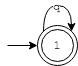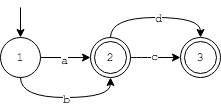edges = {
(1,'q'):1
}
acpt = 

# test-case
print fsmsim("",1,edges,acpt) #True
print fsmsim("q",1,edges,acpt)#True
print fsmsim("qq",1,edges,acpt)#True
print fsmsim("p",1,edges,acpt)#False



edges = {
(1,'a'):2,
(1,'b'):2,
(2,'c'):3,
(2,'d'):3
}
acpt = [2,3]
#test-case
print fsmsim("a",1,edges,acpt)#True
print fsmsim("b",1,edges,acpt)#True
print fsmsim("e",1,edges,acpt)#False



### NFA

Python的re库对正则表达式解析和fsmsim类似，但是上面的fsmsim函数只是实现DFA，没有考虑NFA，具体来说有下面两种情况没有考虑

1. Ambiguity
2. $\epsilon$ 状态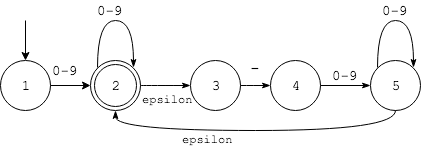1. 从状态起始点#1开始，输入字符为1，走到状态#2
2. 由于有$\epsilon$ 状态，#2可以直接转化为状态#3
3. 状态#3读入-进入状态4
4. 状态#4读入2进入状态5
5. 状态#5读入3之后，产生Ambiguity，一种可能是回到状态#2，一种可能是停留在#5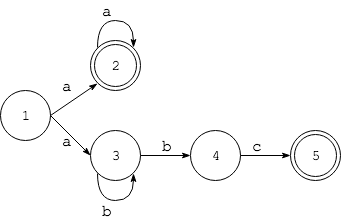edges = { (1, 'a') : [2, 3],
(2, 'a') : ,
(3, 'b') : [4, 3],
(4, 'c') :  }
accepting = [2, 5]

def nfsmsim(string, current, edges, accepting):
if(string==""):
return current in accepting
else:
letter = string
key = (current,letter)
if key in edges:
next_states = edges[key]
for state in next_states:
remain_str = string[1:]
#只有当nfsmsim为true 才返回，false继续尝试
if nfsmsim(remain_str,state,edges,accepting):
return True

return False

# test case
print "Test case 1 passed: " + str(nfsmsim("abc", 1, edges, accepting) == True)
print "Test case 2 passed: " + str(nfsmsim("aaa", 1, edges, accepting) == True)
print "Test case 3 passed: " + str(nfsmsim("abbbc", 1, edges, accepting) == True)
print "Test case 4 passed: " + str(nfsmsim("aabc", 1, edges, accepting) == False)
print "Test case 5 passed: " + str(nfsmsim("", 1, edges, accepting) == False)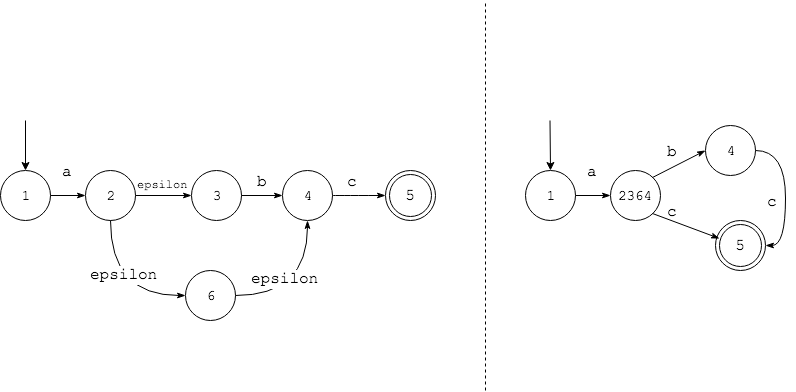### 小结

1. string是一组字符的合集
2. 每个Regular Expression对应一个DFA，反之亦然
3. NFA可以转化为DFA
4. 使用fsmsim函数来实现regular expression的解析

## 词法分析器

1. 首先是匹配token的顺序和优先级，比如匹配WORD的正则式为r'[^ <>]+'，匹配string的正则式为r'"[^"]+"'，对于下字符hello "world",如果WORD的正则在前，则匹配的结果为 [WORD, WORD]，如果STRING在前，则匹配的结果为[WORD, STRING]。
2. 另一问题是不同状态机的互斥，例如，代码注释可以穿插在代码中，对注释的解析需要令一个的状态机，和解析HTML Token的状态机互斥，例如下面的代码
 webpage = '''Welcome to <b>my <!-- careful </b> --> webpage</b>'''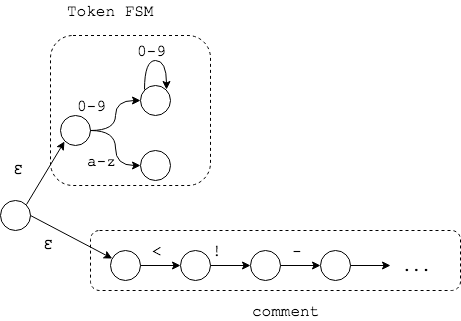### HTML Lexer

tokens = (
'LANGLE', # <
'LANGLESLASH', #</
'RANGLE', #>
'EQUAL', #=
'STRING', #"hello"
'WORD' #welcome!
)


# HTML Tokens
def t_LANGLESLASH(token):
r'</'

def t_LANGLE(token):
r'<'
#...

def t_newline(token):
r'\n'
token.lexer.lineno += 1
pass



htmllexer = lex.lex()
htmllexer.input(webpage)
while True:
#return next token
tok = htmllexer.token()
if not tok:
break
print(tok)



LexToken(WORD,'This',1,0)
LexToken(WORD,'is',1,5)
LexToken(LANGLE,'<',2,26)
LexToken(WORD,'b',2,27)
LexToken(RANGLE,'>',2,28)
LexToken(WORD,'my',2,29)
LexToken(LANGLESLASH,'</',2,31)
LexToken(WORD,'b',2,33)
LexToken(RANGLE,'>',2,34)
LexToken(WORD,'webpage!',2,36)